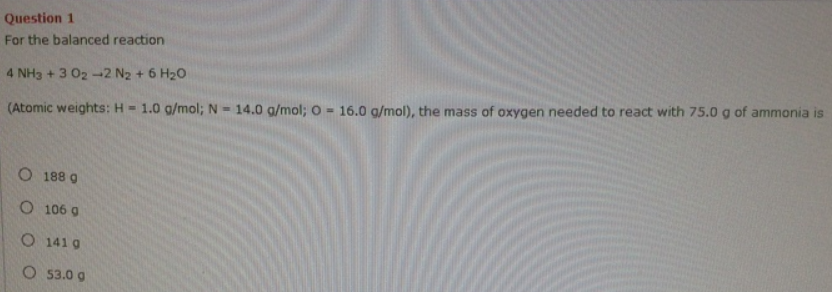# Problem: For the balanced reaction 4NH3 + 3O2 → 2N2 + 6H2O (Atomic weights: H = 1.0 g/mol: N = 14.0 g/mol: O = 16.0 g/mol), the mass of oxygen needed to react with 75.0 g of ammonia is (a) 188 g (b) 106 g (c) 141 g (d) 53.0 g

###### FREE Expert Solution
81% (321 ratings)###### Problem Details

For the balanced reaction

4NH3 + 3O2 → 2N2 + 6H2

(Atomic weights: H = 1.0 g/mol: N = 14.0 g/mol: O = 16.0 g/mol), the mass of oxygen needed to react with 75.0 g of ammonia is

(a) 188 g

(b) 106 g

(c) 141 g

(d) 53.0 g